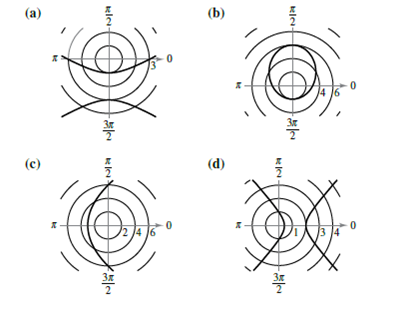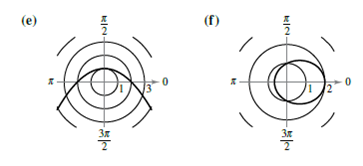Chapter 10.6, Problem 9E

Chapter
Section
Textbook Problem

# Matching In Exercises 7-12, match the polar equation with its correct graph. [The graphs are labeled (a), (b), (c), (d>, (e), and (f).]r = 3 1 − 2 sin θ

To determine

The correct match of the given polar equation with its correct graph from the options given.

Explanation

Given: Given options for graphs are labelled as (a), (b), (c), (d), (e) and (f)as shown below.

Calculation: The given equation is r=312sinθ.

Since e=2>1, the graph of the given equation is a hyperbola.

Because d=32, the directrix is the line y=32 and the transverse axis of the hyperbola lies on the line θ=π2. Also, vertices are at (3,π2) and (1,3π2)

### Still sussing out bartleby?

Check out a sample textbook solution.

See a sample solution

#### The Solution to Your Study Problems

Bartleby provides explanations to thousands of textbook problems written by our experts, many with advanced degrees!

Get Started

#### Find the remaining trigonometric ratios. 34. csc=43,322

Single Variable Calculus: Early Transcendentals, Volume I

#### Expand each expression in Exercises 122. (y1y)2

Finite Mathematics and Applied Calculus (MindTap Course List)

#### A sample with a mean of M = 8 has X = 56. How many scores are in the sample?

Essentials of Statistics for The Behavioral Sciences (MindTap Course List)

#### 2. Define the terms population, sample, parameter and statistic.

Statistics for The Behavioral Sciences (MindTap Course List)

#### Solve the equations in problem 1-6. 2.

Mathematical Applications for the Management, Life, and Social Sciences

#### In Exercises 1-22, evaluate the given expression. C(9,3)

Finite Mathematics for the Managerial, Life, and Social Sciences

#### limx2x2(x1)1x = _____. a) 4 b) 1 c) 0 d) does not exist

Study Guide for Stewart's Single Variable Calculus: Early Transcendentals, 8th

#### The quadratic approximation for at a = 1 is:

Study Guide for Stewart's Multivariable Calculus, 8th